Shell ScriptingLearn About Functions in Linux Shell Scripting

# Learn About Functions in Linux Shell Scripting

There will be cases wherein your need to execute a block of code that achieves a specific procedure several times in different places in your shell script. For these cases, Functions are written. A Function is a block of code that achieves a specific task, and called on need.

Syntax:

```function function_name
{
statement1
statement2
...
statementn
}
```

Where:
function is a key work that declares the function definition.
function_name is the name of the declared function.
The curly braces { } acts as delimiters that enclose the function’s code.
statement1 through n are the code to be executed when the function is called.

So, a function is declared first, and then called when needed.

Example
In this example, we are going to write a function that draws a line consisting of the ‘=’ character.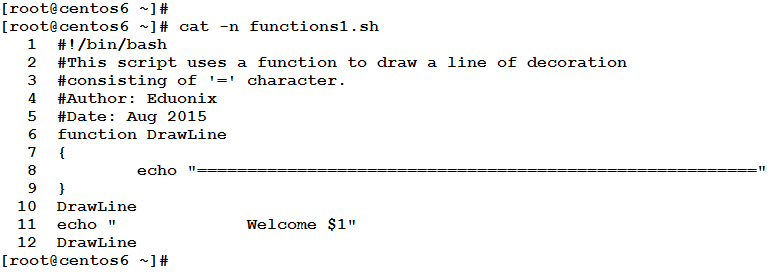Let’s see it in action.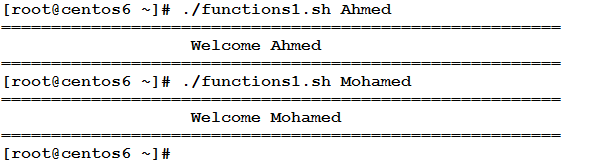Now, to the explanation:

• Line 6: declares the function DrawLine.
• Lines 7 and 9: the curly braces { } that delimit the function body.
• Line 8: the only statement in the function body: uses the echo command to print a line of ‘=’ character.
• Line 10: calls the DrawLine function.
• Line 11: prints the provided argument \$1.
• Line 12: calls the DrawLine function again.

Passing Arguments to Functions
Functions can take arguments. In this case, the syntax will remain the same, but when calling the function; its name will be followed by the argument(s) passed.

Inside the function code block, the arguments from 1 to n are accessed using the positional variables \$1, \$2 . . . , \$n

Example
In this example, we are going to write a function that estimates the length of a string. The string will be provided to the function as an argument. Let’ see how this will look like.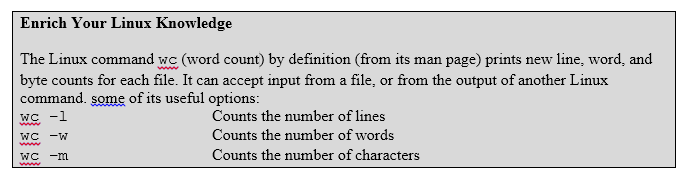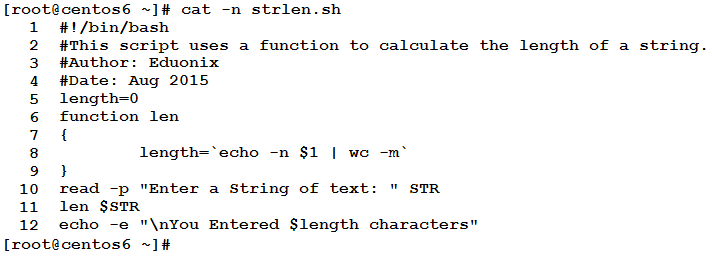How would this script behave?! Let’s watch.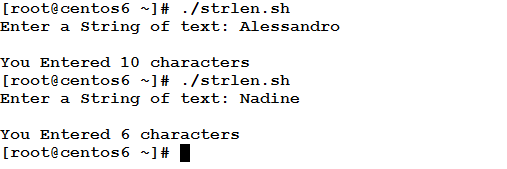Now, to the explanation:

• Line 5: declares and initializes the variable length.
• Line 6: defines the function len.
• Lines 7 and 9: the function code block delimiters.
• Line 8: the only line of code inside the function:
`length=`echo -n \$1 | wc -m``
• The argument passed to the function \$1 is printed using the echo command with the option –n that prevents echo from inserting newline character at the end.
• The output of the command echo –n \$1 is piped into the input stream of the command wc –m (which counts the characters in its input).
• The result of the above composite statement echo -n \$1 | wc -m is assigned (by using backticks ‘ ‘) to the variable length.
• Line 10: reads an input string from the user, and assigns it to the variable STR.
• Line 11: calls the len function and passes the variable STR to it. The result of calling the function is estimating the length of STR, and storing the calculated length in the variable length (as discussed above in Line 8).
• Line 12: prints the result to the user.

Great!!!!Recursive Function Calls
A function can “recursively” call itself. This means within a function definition, and inside the function’s code, a call statement can appear calling the function (being defined) itself. This should be controlled by a test condition, in order to assure the function will converge. If no condition specified, or the wrong one is used, the function will keep calling itself forever. So, take care!!!

Example
Again with our old faithful friend: the factorial example. Now, it comes in a fifth version using the recursive function calls. How would this look like?! To know, read the following script.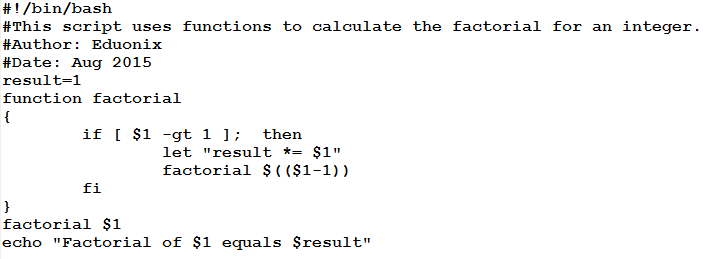Will that achieve the task successfully?! Let’s see in action to know: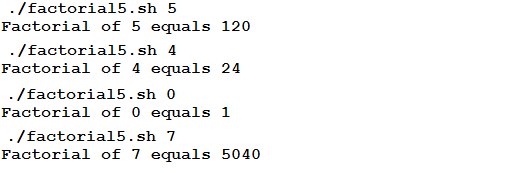– Ah, it works!!!

Yes, sure!! Now, let’s explain it:

• result=1

This line initializes the variable result to 1.

• function factorial

declares the factorial function.

• The function body Staring delimiter {
• if [ \$1 -gt 1 ];  then

checks whether the argument provided to the function is greater than 1. If so, the following two lines are executed:

• let “result *= \$1”

This multiplies the current value of result by the argument passed to the function.

• factorial \$((\$1-1))

This calls the factorial function recursively, and passes to it \$1-1 as an argument.

• The function body closing delimiter }

After the function has been declared, this is again the main script code:

• factorial \$1

The main script calls the factorial function and passes to it the command line argument passed to the script \$1. Don’t confuse the \$1 that represents the first command-line argument, and the \$1 that represents the first argument passed to a function.

• The Last line prints the result to the user.

Now, let’s follow the execution flow of the script when the user executes it using a command-line parameter 4
./factorial5.sh 4

1. First, the result variable is set to 1.
2. The interpreter encounters the function definition.
3. The function is called with an argument 4. The control is transferred to the function that works as follows:

a) The argument (which is now 4) is checked if it is greater than 1 (which is case). If so, the following two lines are executed:

i) The usual accumulative multiplication operation is done: result is multiplied by the argument \$1, and the result is stored in the variable result.
ii) The factorial function is called “recursively”, and passed 3 as an argument. The control is transferred again to factorial function whose argument now is 3.

b) The argument “3” is checked if it is greater than 1 (which is case). If so, the above two steps (i and ii) are executed. The step ii again calls the factorial function with 2 as an argument.
c) This continues until the factorial function is called with argument 1. At this time, the if condition fails, and the function is terminated.

4. The control is back to the main script, and the first line after the function call is executed (which is the echo command that prints the result to the user).

Perfect, isn’t it?!

* * * * * *

In this article, we have talked about Functions in Linux shell scripts. A Function is a block of code that achieves a specific task, and called on need. Functions can take argument(s), or called without arguments at all. We have illustrated our talk with examples. I hope you find this article useful.

### Top 10 cyber security threats that arose last year

21,501Fans
4,218Followers
103,000Subscribers

### Top 10 cyber security threats that arose last year

- Advertisement -